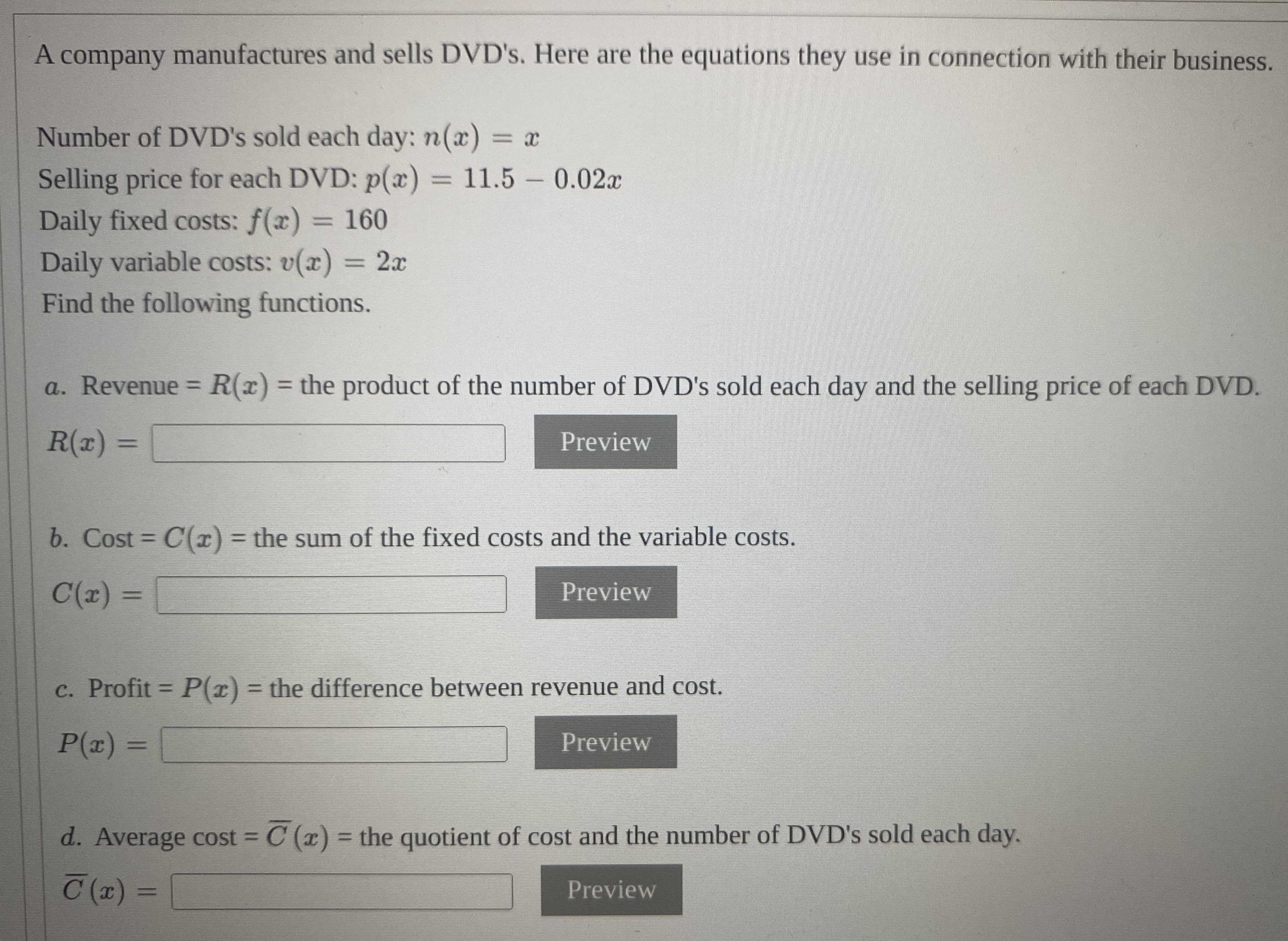### ¿Todavía tienes preguntas de matemáticas?

Pregunte a nuestros tutores expertos
Algebra
PreguntaA company manufactures and sells DVD's. Here are the equations they use in connection with their business.

Number of DVD's sold each day: $$n ( x ) = x$$

Selling price for each DVD: $$p ( x ) = 11.5 - 0.02 x$$

Daily fixed costs: $$f ( x ) = 160$$

Daily variable costs: $$v ( x ) = 2 x$$

Find the following functions.

a. Revenue $$= R ( x ) =$$ the product of the number of DVD's sold each day and the selling price of each DVD.

$$R ( x ) = \square$$

b. Cost $$= C ( x ) =$$ the sum of the fixed costs and the variable costs.

$$C ( x ) = \square$$

c. Profit $$= P ( x ) =$$ the difference between revenue and cost.

$$P ( x ) = \square$$

$$d$$ . Average cost $$= \overline { C } ( x ) =$$ the quotient of cost and the number of DVD's sold each day.

$$\overline { C } ( x ) = \square$$